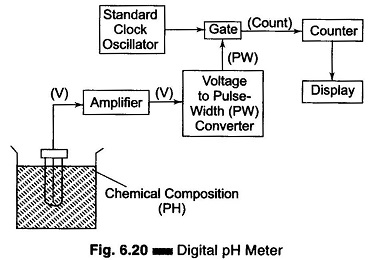## Working Principle of Digital pH Meter | Block Diagram:

Working Principle of Digital pH Meter – The Digital Measurement of hydrogen ion activity (pH) in a solution can be accomplished with the help of a pH meter. For those unfamiliar with the terminology, a very brief review is included.

pH is a quantative measure of acidity. If the pH is less than 7, the solution is acidic (the lower the pH, the greater the acidity). A neutral solution has a pH of 7 and alkaline (basic) solutions have a pH greater than 7.

The pH unit is defined as

pH = – log (concentration of H+)

where

• H+ is the hydrogen or hydronium ion.

A digital pH meter differs from an ordinary pH meter, in this the meter is replaced by an analog to digital converter (ADC) and a digital display. A frequently used ADC for this application is the dual slope converter. A basic block diagram of a digital pH meter is shown in Fig. 6.20.The dual slope circuit produces a pulse which has a duration proportional to the input signal voltage, that is, a T pulse width signal. The pulse width is converted to a digital signal using the pulse to turn an oscillator On or Off, generating a count digital signal. The count signal is in turn counted or converted to a parallel digital signal for display by the counter.

Scroll to Top